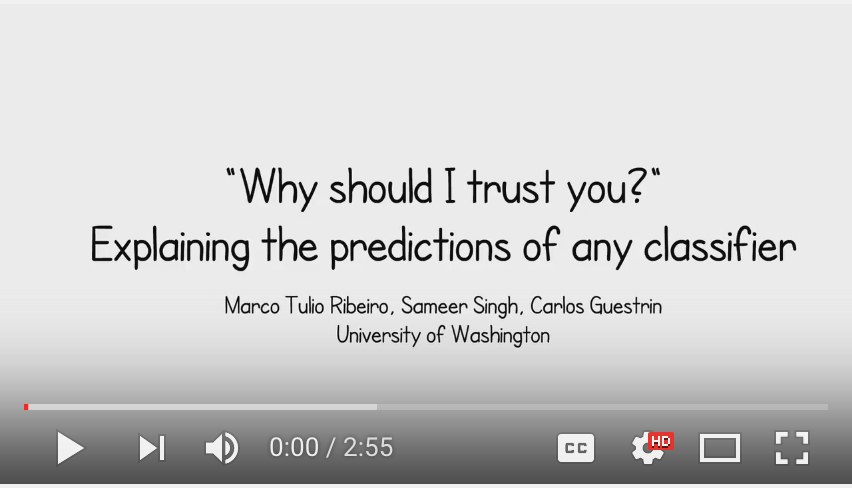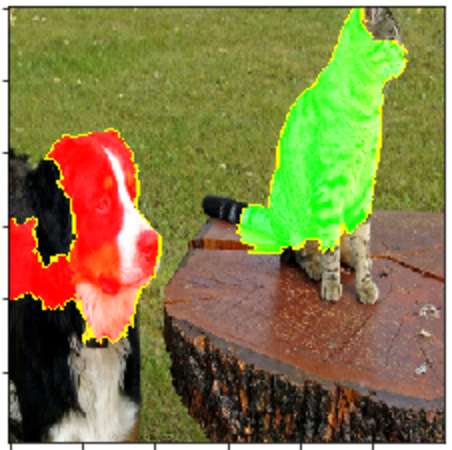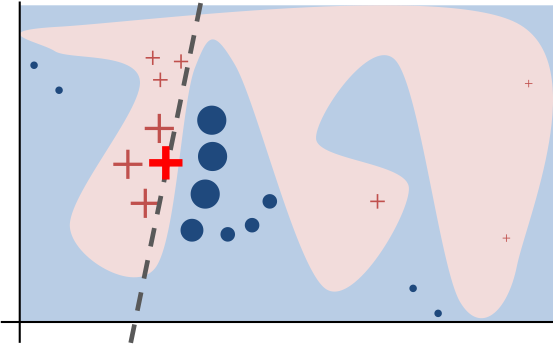# lime

This project is about explaining what machine learning classifiers (or models) are doing. At the moment, we support explaining individual predictions for text classifiers or classifiers that act on tables (numpy arrays of numerical or categorical data) or images, with a package called lime (short for local interpretable model-agnostic explanations). Lime is based on the work presented in this paper (bibtex here for citation). Here is a link to the promo video:Our plan is to add more packages that help users understand and interact meaningfully with machine learning.

Lime is able to explain any black box classifier, with two or more classes. All we require is that the classifier implements a function that takes in raw text or a numpy array and outputs a probability for each class. Support for scikit-learn classifiers is built-in.

## Installation

The lime package is on PyPI. Simply run:

`pip install lime`

Or clone the repository and run:

`pip install .`

We dropped python2 support in `0.2.0`, `0.1.1.37` was the last version before that.

## Screenshots

Below are some screenshots of lime explanations. These are generated in html, and can be easily produced and embedded in ipython notebooks. We also support visualizations using matplotlib, although they don’t look as nice as these ones.

#### Two class case, text

Negative (blue) words indicate atheism, while positive (orange) words indicate christian. The way to interpret the weights by applying them to the prediction probabilities. For example, if we remove the words Host and NNTP from the document, we expect the classifier to predict atheism with probability 0.58 – 0.14 – 0.11 = 0.31.#### Multiclass case#### Tabular data#### Images (explaining prediction of ‘Cat’ in pros and cons)## Tutorials and API

For example usage for text classifiers, take a look at the following two tutorials (generated from ipython notebooks):

For classifiers that use numerical or categorical data, take a look at the following tutorial (this is newer, so please let me know if you find something wrong):

For image classifiers:

For regression:

Submodular Pick:

The raw (non-html) notebooks for these tutorials are available here.

The API reference is available here.

## What are explanations?

Intuitively, an explanation is a local linear approximation of the model’s behaviour. While the model may be very complex globally, it is easier to approximate it around the vicinity of a particular instance. While treating the model as a black box, we perturb the instance we want to explain and learn a sparse linear model around it, as an explanation. The figure below illustrates the intuition for this procedure. The model’s decision function is represented by the blue/pink background, and is clearly nonlinear. The bright red cross is the instance being explained (let’s call it X). We sample instances around X, and weight them according to their proximity to X (weight here is indicated by size). We then learn a linear model (dashed line) that approximates the model well in the vicinity of X, but not necessarily globally. For more information, read our paper, or take a look at this blog post.